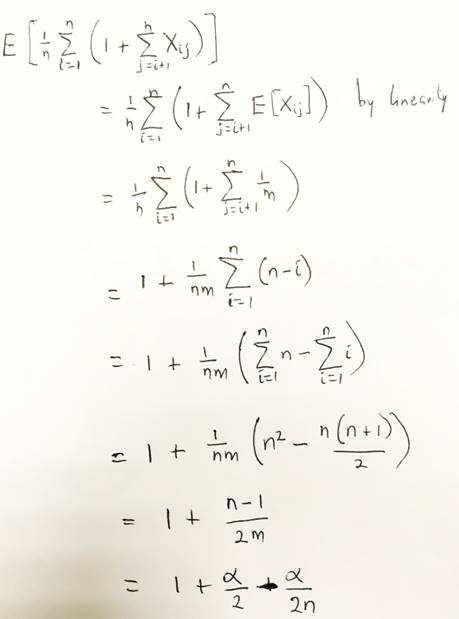# Search Time Under Simple Uniform Hashing

We are going to examine the time it takes for successful and unsuccessful search in a hash table where collision is resolved by chaining under Simple Uniform Hashing.

We would state the Theorem and the try to prove it.

##### 1.  Unsuccessful Search

In a hash table where collision is resolved by chaining, and simple uniform hashing is assumed, then an unsuccessful search takes and average of Θ(1 + α)

Proof: In simple uniform hashing is assumed, then any key k that is not already in the hash table would have equal likelihood of being hashed to any of the m slots in the table. The expected time to search unsuccessfully for a key is the same as the expected time to search from through the length of the list T[h(k)]. And expected length of the list is given as α or the load factor of the table. Therefore, the expected number of elements to check  in an unsuccessful search is α.  If we add to time it takes to compute the hash function we would have a total expected time of Θ(1 + α)

##### 2. Successful Search

In a hash table where collision is resolved by chaining, and simple uniform hashing is assumed, then the expected time for a successful search is given as Θ(1 + α).
Proof: In this case where the search is successful, all the items in the list is not equally likely to be searched. The probability that a list is searched is proportional to the number of elements contained in the list

So, we assume that the element being searched  for could equally be any of the n elements stored in the table. If the element being searched for is x, then the number of elements checked during the search is one more than the number of elements that appear before x in the linked list containing x. Since new elements are placed in front of a linked list, elements before x in the list were all inserted after x was inserted.

###### Expected Number of Elements

To get the expected number of elements examined, we would take an average  over the n elements in the list  and 1 plus the expected number of elements inserted into x’s list after x have been inserted.

Let xi be the ith element inserted into the table, for i = 1, 2, . . . , n and ki = xi.key. We would then use an indicator random variable to iterate through the keys  to check if there is a match. Let For keys ki and kj, we define an indicator random variable:

Xij = I{h(ki) = h(kj)}

Under simple uniform hashing, we would have that:

Pr{h(ki) = h(kj)} = 1/m

We can then find this expectation as:

E[Xij] = 1/m

This is therefore we can find the expected number of elements examined in a successful search using the derivation given below:Finally we have the total time required for a successful search (which includes the time it takes to compute the hash function )  as

Θ(2 + α/2 – α/2n) = Θ(1 + α)

This means that if the number of slots in the hash table is at least proportional to the number of elements stored in the table then we have n = O(m) and it follows that α = n/m = O(m)/m = O(1).

Therefore, this we can see that searching takes constant time on the average. Since insertion of item takes O(1) time in the worst-case, and deletion takes O(1) time in the worst-case, it means that all the operations can be done in O(1) on average assuming simple uniform hashing.

##### Check these Data Structure and Algorithms Tutorials#### kindsonthegenius

Kindson Munonye is currently completing his doctoral program in Software Engineering in Budapest University of Technology and Economics

View all posts by kindsonthegenius →